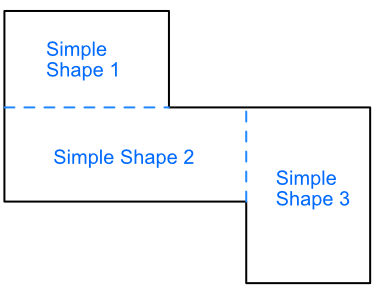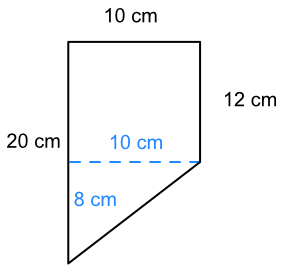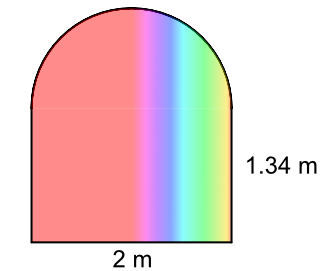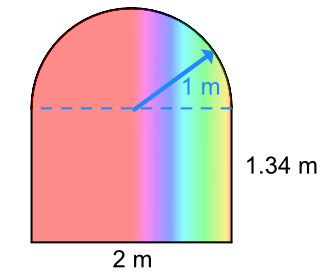Areas of Composite Shapes

## Areas of Composite Shapes

A compound shape is a shape that is built up from two or more simple shapes. A simple shape is a shape for which a formula for calculating area is readily available, such as triangles, rectangles and circles.

To calculate the area of a complex shape, divide the complex shape into a number of simple shapes. There are often a number of ways to divide the shape up: it does not matter which combination is used so long as the whole complex shape is taken into account.

The area of each simple shape is calculated separately; then added together as a total for the compound shape.## Example 1

What is the area of this shape?Split the compound shape into two simple shapes: a rectangle and a triangle:The area of the rectangle part is 12 x 10 = 120 cm2.

The missing dimension for the triangle part is 20 - 12 = 8cm.

 Area of a triangle A = frac(1)(2)bh Substitute: = frac(1)(2) xx 10 xx 8 = 40 cm2.

Area of compound shape

= area of rectangle + area of triangle

= 120 + 40

= 160 cm2.

## Example 2

A stained glass window is being manufactured as the centrepiece of a new home. The stained glass will cost £250 per square metre. How much will the stained glass cost for the window?The lower part of the window is a rectangle:

 Area of a rectangle A = b xx h Substitute: = 2 x 1.34 = 2.68 cm2.

The upper part of the window is a semicircle (half a circle).

The diameter of the semicircle is the width of the window;

the radius is half of that = 1m.

 Area of a whole circle A = πr^2 Area of a semicirclee A = frac(1)(2) xx πr^2 Substitute: = frac(1)(2) xx π xx 1^2 = 4.25 cm2.

Area of entire window = 2.68 + 1.57 = 4.25 m2

Cost of window = 250 x 5.82 = £1062.50